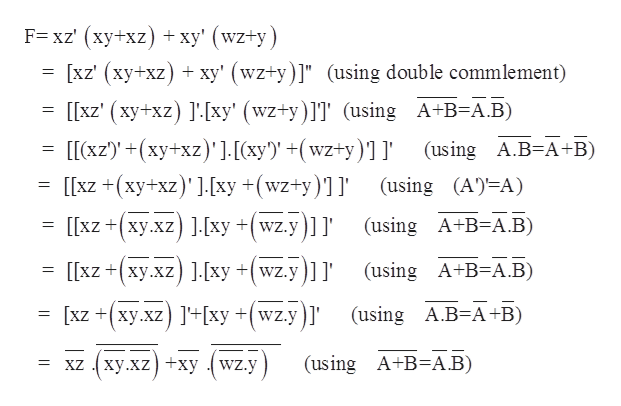# Using DeMorgan's Law, write an expression for the complement of F ifF(x,y,z) = xz' (xy + xz)+ xy'(wz + y)

Question
1 views

Using DeMorgan's Law, write an expression for the complement of F if
F(x,y,z) = xz' (xy + xz)+ xy'(wz + y)

check_circle

Step 1

Expressio...help_outlineImage TranscriptioncloseF= xz' (xy+xz +xy' (wz+y) xz' (xy+xz) xy' (wz+y)]" (using double commlement) [[xz' (xy+xz) ]'[xy' (wz+y)]]' (using A+B=A.B) [[(xz)+(xy+xz)]. [(xy')' +(wz+y)]I' [[xz +(xy+xz).[xy +(wz+y)]] (using A.B=A+B) (using (A A) [[xz xy.xz ].[xy +(wz.y]]' (using A+B-A.B) (using A+B A.B) [[xzxy.xz 1[xy +wz.y]]' (using A.B A +B) xz xy.xz ]'+[xy +(wz.yl' (using A+B A.B) xZxy.xz xy wz.y fullscreen

### Want to see the full answer?

See Solution

#### Want to see this answer and more?

Solutions are written by subject experts who are available 24/7. Questions are typically answered within 1 hour.*

See Solution
*Response times may vary by subject and question.
Tagged in

### Boolean Logic